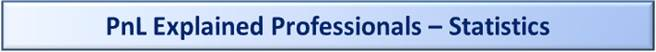An Unbiased Estimator of the Variance

Overview

The purpose of this document is to explain in the clearest possible language why the "n-1" is used in the formula for computing the variance of a sample.

The Mean of a Probability Distribution (Population)

The Mean of a distribution is its long-run average.  Alternately you could say it is the probability weighted average of each possible value.  The symbol for Mean of a distribution is μ.

We could restate the above by using this formula: μ = Σ [(xi) * p(xi)]

• Σ is the symbol meaning "sum up for all values"
• xi is a particular value of x.  If there are n possible values then "i" is every integer between 1 and n inclusive.   So if n is 3 then "i" would be [1,2,3] and all of the xi values would be [x1, x2, x3].
• * is the symbol for multiplication
• p(xi) is the probability of xi occurring.
• In the case of the die roll, the formula would be written out the long way as: (1 * 1/6) + (2 * 1/6) + (3 * 1/6) + (4 * 1/6) + (5 * 1/6) + (6 * 1/6) = 3.5

Further Notes On This Formula:

• If there is an equal probability of all items occurring then you can write the formula as: μ = [Σ(xi)] / n, which for the die example would be (1+2+3+4+5+6)/6 = 3.5.
• This formula can only be used if you are dealing with a discrete probability distribution (like a die roll).  There are other analogous ways to find the expected value for a continuous distribution (like the normal distribution).
• This formula can only be used if you have precise knowledge of every possible value (every possible xi) and also know the exact probability of each occurrence.
• Some probability distributions have well understood properties and have shortcut formulas for the Mean.  For example, the formula for the Mean for the Binomial Distribution is np where n is the sample size and p is the probability for success.  So if n is 10 and p is 0.6 then the expected value is 6.  Whether you use the shortcut formula or the long way you'll get the same Mean.
• Do not confuse this formula with the formula for the sample mean, which looks similar at first glance.  The sample mean is a random variable with a well-understood distribution.  The Mean is not a random variable; it is a single number that can be calculated with 100% certainty for any distribution in which you know all values and probabilities.

The Sample Mean Of A Sample Taken From A Probability Distribution

The Sample Mean from a distribution is the probability weighted average of each sample.   Typically we assume we are dealing with an unbiased sample, which means that we are assuming that the probability of each sample occurring is 1/n where n is the number of the sample.  So if you roll the die 8 times, your sample size (your n) is 8 and the probability of each sample is .  The symbol for the Sample Mean is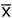.

We could restate the above by using this formula:= Σ [(xi) * 1/n]

• We could also write the above formula as= Σ(xi)/n
• Σ is the symbol meaning "sum up for all values"
• n is the sample size
• xi is a particular sample value.  If there are n possible values then "i" is every integer between 1 and n inclusive.   So if n is 3 then "i" would be [1,2,3].
• * is the symbol for multiplication
• In the case of a die rolled 3 times then n would be 3.  If the values are [2,3,6] then the sample mean would be:  (2 * 1/3) + (3 * 1/3) + (6 * 1/3) = 3.6666.

Further Notes On This Formula:

• This formula can be used whether the distribution from which you are taking the sample is discrete or continuous.
• Sometimes your Sample Mean will equal the Expected Value of the distribution.  This does not need to be the case so if it does happen it is typically just a coincidence (depending on what the original population looks like).  For example, if you roll the dice twice and you roll a 1 and a 6 then the sample mean would be equal to 3.5 which is coincidentally the Mean of the original distribution (also called the True Mean).

The Variance of a Probability Distribution (Population)

The Variance is the Expected Value of the squared deviations from the mean.

By "deviations from the mean" we are talking about (xi - μ) where xi is a single particular sample from a distribution and μ is the mean of the distribution.  If we think about the roll of a single die then xi might be 1 though 6 and μ is 3.5.  In the die roll example, since there are only 6 possible values there are also 6 possible deviations from the mean.  They are:

 1-3.5 = -2.5 2-3.5 = -1.5 3-3.5 = -0.5 4-3.5 = +0.5 5-3.5 = +1.5 6-3.5 = +2.5

By "squared deviation from the mean" we are talking about the previous set of numbers squared.  Squaring the number has the beneficial affect of making every number positive.  Without squaring the numbers, then the expected value of the deviation of the mean would be zero.  In other words, the average of -2.5, -1.5, -0.5, +0.5, +1.5, +2.5 is zero.  Here are the numbers for the die roll example squared.

 (1-3.5)2 = (-2.5)2 = 6.25 (2-3.5)2 = (-1.5)2 = 2.25 (3-3.5)2 = (-0.5)2 = 0.25 (4-3.5)2 = (+0.5)2 = 0.25 (5-3.5)2 = (+1.5)2 = 2.25 (6-3.5)2 = (+2.5)2 = 6.25

By "Expected Value" we mean the long run average.   It is the probability weighted average of the values.  For the die roll example, in the long run each side will have a 1/6th chance of appearing.  So the expected value of the squared deviations of the mean is (6.25 * 1/6) + (2.25 * 1/6) + (0.25 * 1/6) + (0.25 * 1/6) + (2.25 * 1/6) + (6.25 * 1/6) = 2 11/12 = 2.91666.

In the simple case where every xi has the same probability you could write this as (6.25 + 2.25 + 0.25 + 0.25 + 2.25 + 6.25) /6.

The Variance is denoted using this symbol: σ2

Expected Value is denoted by this symbol: E(x)

You could write the expected value of the squared deviation of the mean like this:

σ2 = E[(xi - μ)2]

You could also write it like this:

σ2 = Σ[(xi - μ)2 * p(xi)]

Σ is a symbol meaning to sum all values.  You would sum all values from "i" equals 1 to n, where n is the total number of values.

* just means to multiply

p(x) is the probability that a particular x will occur.

We applied this formula for the die roll example above (repeated here):

((1-3.5)2  * 1/6) + ((2-3.5)2 * 1/6) + ((3-3.5)2 * 1/6) + ((4-3.5)2 * 1/6) + ((5-3.5)2 * 1/6) + ((6-3.5)2 * 1/6) = 2.916666.

For the purposes of this document, we'll only be looking at cases where the probability of each occurrence is equal.   So for the rest of the document we'll be using a slightly simpler version of the above formula than can only be used if the probability of each occurrence is equal.  Again, we are using this because it is simpler to read and understand and it is all we'll need.

The variation on the formula is:

σ2 = Σ[(xi - μ)2] / n

You can also write the above formula like this:

σ2 = Σ(xi2)/n - μ2

See Appendix A for a derivation on this alternate form of the formula.

In the special case of each xi having the probability of 1/n (meaning p(xi) is 1/n for all "i") you can use this formula:

σ2 = [Σ(xi2) - nμ2] / n

σ2 = Σ(xi2)/n - μ2

For the die roll example, that would be:

Step 1) (12 + 22 + 32 + 42 + 52 + 62)/6 - 3.52

Step 2) (1 + 4 + 9 + 16 + 25 + 36)/6 - 12.25

Step 3) (91/6) - 12.25

Step 4) 15.16666 - 12.25 = 2.916666

Note: In this case we have a probability distribution that has an equal probability for each possibility (for each x).  That is just a coincidence.  Later we will look at samples from a distribution (with the die roll example we would be talking about rolling a single die typically two or more times to get sample).  In general, no matter what main population looks like, we will assume samples from that population are equally likely, that each sample has an equal probability of occurring (this is synonymous with saying our sampling is unbiased).

The Variance of a Sample from a Probability Distribution

The formula for the variance of a sample taken from a Probability Distribution is:

s2 = Σ[(xi -)2] / n

• s2 is the symbol for the variance of a sample.
• n is the sample size
• xi is a particular sample value.  If there are n possible values then "i" is every integer between 1 and n inclusive.   So if n is 3 then "i" would be [1,2,3].
•is the sample mean calculated using this formula:= Σ(xi)/n

Important Note: Σ[(xi - μ)2]    Σ[(xi -)2]

Why?  The main reason is that the sample mean() is not equal to the "true" mean(μ) of a population (≠ μ).

μ is the true population mean and is constant number that can be computed when you know all of the possible xi values.is the sample mean for a particular sample of size n.  It is a random variable that has an expected value of μ and a standard deviation that is related to the standard deviation of the population by this formula:

σ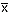= σx / n

The sample mean is normally a random variable with a particular mean and variance of its own.  However, when used in the context of the s2 formula (Σ[(xi -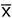)2] / n), the sample mean should not be thought of as a random variable at all.  It is completely determined by the xi values and should not even be though of as a separate variable.  In fact, you can rewrite the formula to get rid of theterm entirely by replacing it with the formula used to calculate it.

I.e., s2 = Σ[(xi - (Σ(xi)/n))2] / n)

For example, when n equals 2, this becomes:

s2 = Σ[(xi - (x1 + x2)/2))2] / 2)

which is equivalent to this:

s2 = [(x1  -)2 + (x2  -)2] / 2

You can further reduce this to this (when n = 2):

s2 = (½x1  - ½x2)2

See Appendix B for more details.

So the question is, if s2 = Σ[(xi -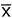)2]/n then what is the expected value of s2 or what is E(s2)?  If it is equal to σ2 then it is an unbiased estimator of σ2.   As it turns out, s2 is not an unbiased estimator of σ2.

First lets write this formula:

s2 = Σ[(xi -)2] / n

like this:

s2 = [ Σ(xi2) - n2  ] / n

(you can see Appendix A for more details)

Next, lets subtract μ from each xi. This will leave s2 unchanged as long as we also subtract it from.

s2 = [ Σ(xi2) - n2  ] / n

and get this:

s2 = [ Σ(xi - μ)2 - n(- μ) 2 ] / n

(See Appendix C for details)

Here we'll find the expected value of s2:

Step 1) s2 = Σ[(xi -)2] / n

This is the starting point.

Step 2) ns2 = Σ[(xi -)2]

Multiply both sides by n to make the formulas easier to read:

Step 3) ns2 = Σ[(xi - μ -+ μ)2]

Add and subtract μ, the population mean.  Notice that adding and subtracting any number nets to zero, so this is ok.

Step 4) ns2 = Σ[(xi - μ)2] - n(- μ)2

The right side term is shown to be the same as the formula of s2 in Appendix C.  Or you could say Σ[(xi - μ)2] - n(- μ)2  = Σ[(xi -)2] is proven in Appendix C.

Step 5) E(ns2) = nσ2 - n(- μ)2

Replace Σ[(xi - μ)2] with nσ2.

Why?  By definition σ2 = E[(xi - μ)2], which equals Σ[(xi - μ)2]/n when the probability of each xi is identical, which is the case as we are assuming each sample has the same probability.

So if σ2 = Σ[(xi - μ)2]/n then nσ2 = Σ[(xi - μ)2] so we are able to replace this term in the equation.

Step 6) E(ns2) = nσ2 - Σ[(- μ)2]

Since n(- μ)2 = Σ[(- μ)2]

Step 7) E(ns2) = nσ2 - n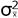Replace Σ[(- μ)2] with nWhy?  If σ2 = E[(xi - μ)2] then= E[(- μ)2]

If σ2 = Σ[(xi - μ)2]/n then= Σ[(- μ)2]/n  (when the probability of each item is equal.)

If= Σ[(- μ)2]/n then multiply both sides by n to get n= Σ[(- μ)2]

Step 8) E(ns2) = nσ2 - σ2

Replace n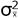withWhy?  We have seen previously that= σ2/ n.  That is, the variance of the sample mean is equal to the variance of the original probability distribution divided by n, where n is the sample size.

Since= σ2/n then σ2 = nStep 9) E(ns2) = (n-1) σ2

Factor out the n-1.

Step 10) E(s2) = (n-1) σ2/ n

Divide both sides by n.

Therefore the expected value of s2 is not σ2.  To get an unbiased estimator use this:

s2 = Σ[(xi -)2]/(n-1) instead since E(Σ[(xi -)2]/(n-1)) = σ2

Appendix A

Going from this:

σ2 = Σ[(xi - μ)2] / n

to this:

σ2 = Σ(xi2)/n - μ2

Summary

Step 1) σ2 = Σ[(xi - μ)2] / n

Step 2) nσ2 = Σ[(xi - μ)2]

Step 3) nσ2 = Σ[(xi - μ) * (xi - μ)]

Step 4) nσ2 = Σ[(xi2 -μxi - μxi + μ2)]

Step 5) nσ2 = Σ[(xi2 - 2μxi + μ2)]

Step 6) nσ2 = Σ(xi2) - Σ(2μxi) + Σ (μ2)

Step 7) nσ2 = Σ(xi2) - [2μ *Σ(xi)] + Σ (μ2)

Step 8) nσ2 = Σ(xi2) - [2μ *Σ(xi)] + nμ2

Step 9) nσ2 = Σ(xi2) - [2μ * )] + nμ2

Step 10) nσ2 = Σ(xi2) - 2nμ2 + nμ2

Step 11) nσ2 = Σ(xi2) - nμ2

Step 12) σ2 = Σ(xi2)/n - μ2

Details

Step 1) σ2 = Σ[(xi - μ)2] / n

This is just the normal formula for variance of a population

Step 2) nσ2 = Σ[(xi - μ)2]

Multiply both sides by n.  The only reason to do this is to make it easier to read.  Our last step is to undo this by dividing both sides by n.

Step 3) nσ2 = Σ[(xi - μ) * (xi - μ)]

Write this out in a longer form.  So instead of writing a2 write a * a

Step 4) nσ2 = Σ[(xi2 -μxi - μxi + μ2)]

Perform the multiplication.  Remember FOIL (First, Outer, Inner, Last)?  So instead of writing (a - b) * (a - b), write: (a2 - 2ab + b2)

Step 5) nσ2 = Σ[(xi2 - 2μxi + μ2)]

This completes the factoring step begun in Step 4.

Step 6) nσ2 = Σ(xi2) - Σ(2μxi) + Σ (μ2)

Move the summation signs next to each value.  You can do this because you are just adding or subtracting each term.  You couldn't do this if you were multiplying or dividing each term.

Step 7) nσ2 = Σ(xi2) - [2μ *Σ(xi)] + Σ(μ2)

Move the 2μ to the outside of the summing of the xi terms.  Why is this OK?  Remember that the Σ symbol means sum all of the terms for all xi for "i" equals 1 to n.  Since the 2 and the μ are constant and therefore unaffected by particular value of the xi, you can move them to the outside of the summation notation.  You wind up multiplying once at the end of the summing rather than multiplying for each loop in the summing process, but you get the same result.

Step 8) nσ2 = Σ(xi2) - [2μ *Σ(xi)] + nμ2

Σ(μ2) becomes nμ2.  Why?  Remember that the Σ symbol specifically means to sum for all values of xi from "i" equals 1 to n .  Since μ is a constant across all values of xi, you can just multiply μ2 by n to get the same result as you would get by summing it n times.

Step 9) nσ2 = Σ(xi2) - [2μ * )] + nμ2

Σ(μ) becomes .  Why?  Same logic as for Step 8.

Step 10) nσ2 = Σ(xi2) - 2nμ2 + nμ2

This is just rewriting the formula to make the middle term easier to read.

Step 11) nσ2 = Σ(xi2) - nμ2

Add the last two terms on the right side of the equation.

Step 12) σ2 = Σ(xi2)/n - μ2

Divide both sides by n to get the result we wanted, the alternate formula for σ2.

Appendix B

This:       [(x1  -)2 + (x2  -)2] / 2

becomes:   (½x1  - ½x2)2

Summary

Step 1) [(x1  -)2 + (x2  -)2] / n

Step 2) [(x1-(x1+x2)/n)2 + (x2-(x1+x2)/n)2] / n

Why?= (x1 + x2 ) / n

Step 3) [(x1-(x1+x2)/2)2 + (x2-(x1+x2)/2)2] / 2

Step 4) [(x1 - (x1/2) - (x2/2))2 + (x2 - (x1/2) - (x2/2))2

Step 5) [(½x1-(x2/2))2+(½x2-(x1/2))2]/2

Step 6) [(½x1-½x2))2+(½x2-½x1))2]/2

Step 7) [(½x1-½x2) * (½x1-½x2) + (½x2-½x1) * (½x2-½x1)]/2

(a - b) * (a - b) = a2 - ba - ba + b2

Step 8) [((½x1)2 - (½ * ½ * x1*x2) - (½ * ½ * x1*x2) + (½x2)2) +

((½x2)2 - (½ * ½ * x2*x1) - (½ * ½ * x2*x1) + (½x1)2)] / 2

Step 9) [((½x1)2 - (1/4x1x2) - (1/4x1x2) + (½x2)2) +

((½x2)2 - (1/4x2x1) - (1/4x2x1) + (½x1)2)] / 2

Step 10) [(½x1)2 + (½x2)2 + (½x2)2 + (½x1)2 - (x1x2)] / 2

Step 11) [¼x12 + ¼x22 + ¼x22 + ¼x12 - (x1x2)] / 2

Step 12) [1/2x12 + 1/2x22 - (x1x2)] / 2

Step 13) (¼x12  - ½x1x2 + ¼x22)

Step 14) (¼x12  - ¼x1x2  - ¼x1x2 + ¼x22)

Step 15) (½x1  - ½x2) * (½x1  - ½x2)

Step 16) (½x1  - ½x2)2

With n = 3 you get:

1) s2 = [(x1  -)2 + (x2  -)2 + (x3  -)2] / n

2) s2 = [(x1-(x1+x2+x3)/n)2 + (x2-(x1+x2+x3)/n)2 + (x3-(x1+x2+x3)2]/ n)2]/n

(Since= (x1 + x2 + x3) / n)

3) s2 = [(x1-(x1+x2+x3)/3)2 + (x2-(x1+x2+x3)/3)2 + (x3-(x1+x2+x3)2]/3)2]/3

5) [(x1 - (x1/3) - (x2/3) - (x3/3))2 + (x2 - (x1/3) - (x2/3) - (x3/3))2 +

(x3 - (x1/3) - (x2/3) - (x3/3))2] / 3

6) [(2/3x1-(x2/3)-(x3/3))2+(2/3x2-(x1/3)-(x3/3))2+(2/3x3-(x1/3)-(x2/3))2]/n

7) The above formula can be reduced further (but not here due to space constraints.)

Appendix C

This:       [ Σ(xi - μ)2 - n(- μ) 2 ] / n

Is equivalent to this:   [ Σ(xi2) - n2] / n

Here are the steps to go from one to the other:

Step 1) s2 = [ Σ(xi - μ)2 - n(- μ) 2 ] / n

Step 2) s2 = [ Σ[(xi - μ) * (xi - μ)] - n[(- μ) * (- μ)] ] / n

Step 3) s2 = [ Σ(xi2 - 2μxi + μ2) - n(2 - 2μ+ μ2) ] / n

Step 4) s2 = [ Σ(xi2) - Σ(2μxi) + Σ(μ2) - n2 + 2nμ- nμ2 ] / n

Step 5) s2 = [ Σ(xi2) - 2μΣ(xi) + nμ2 - n2 + 2nμ- nμ2 ] / n

Step 6) s2 = [ Σ(xi2) - 2μΣ(xi) - n2 + 2nμ] / n

Step 7) s2 = [ Σ(xi2) - 2μn- n2 + 2nμ] / n

Step 8) s2 = [ Σ(xi2) - n2] / n